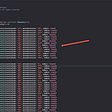# Quick Sort Algorithm

`function pivot(arr, start = 0, end = arr.length - 1) {  const swap = (arr, idx1, idx2) => {    [arr[idx1], arr[idx2]] = [arr[idx2], arr[idx1]];  };  // We are assuming the pivot is always the first element  let pivot = arr[start];  let swapIdx = start;  for (let i = start + 1; i <= end; i++) {    if (pivot > arr[i]) {      swapIdx++;      swap(arr, swapIdx, i);    }  }  // Swap the pivot from the start the swapPoint  swap(arr, start, swapIdx);  return swapIdx;}function quickSort(arr, left = 0, right = arr.length -1){    if(left < right){        let pivotIndex = pivot(arr, left, right) //3        //left        quickSort(arr,left,pivotIndex-1);        //right        quickSort(arr,pivotIndex+1,right);      }     return arr;}`

--

--

--

## More from SunJet Liu

Love podcasts or audiobooks? Learn on the go with our new app.

## CSS Tips : Color text using image## Classes and Objects## final, finally, and finalize in Java. Usage and Examples.## Tutorial Fuzzy Logic Mamdani for Arduino## How to get the Current Country Phone Code?## Spring 2022 Playlist## Dijkstra’s Algorithm## CS371p Spring 2022: Raju Kakarlapudi## CS373 Spring 2022: Martin Nguyen